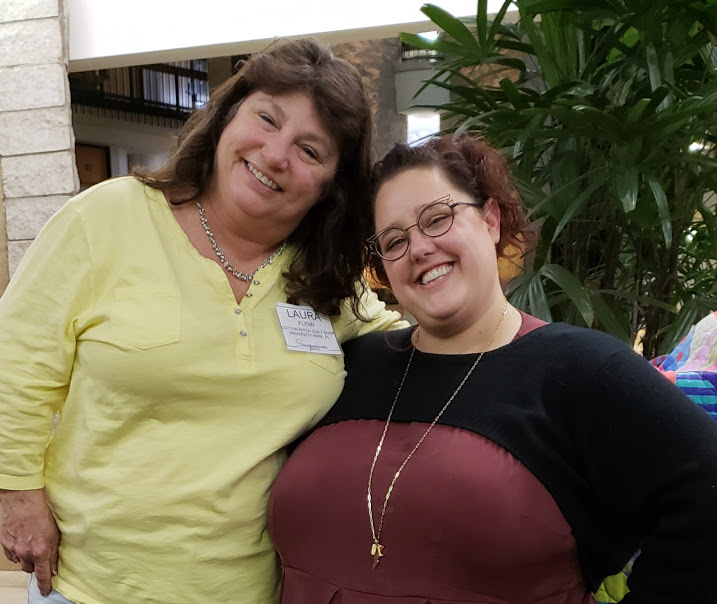Look who I was with this week!

I am spending the week in Orlando at Sewposium, a shop owner retreat and our special guest today was none other than Tula Pink! She gave a fascinating program about her fabric design process and a trunk show of her Zuma quilts.

Did you know that Tula wanted to write and illustrate children's books, never uses the color brown and makes all her own samples?

We have been learning how to be better shop owners, getting exclusive projects - including the Brown Bag Mystery, networking with shop owners around the country and we even get to sew!
Look for a full report next week when I sort out what I learned!

 table div table+table+table div table{width:100%;padding:0}table div table+table+table div table img{width:96.23%;padding:0;float:none}table div table+table+table div table td{width:100%;padding:0 1.88% 18px}/* styles */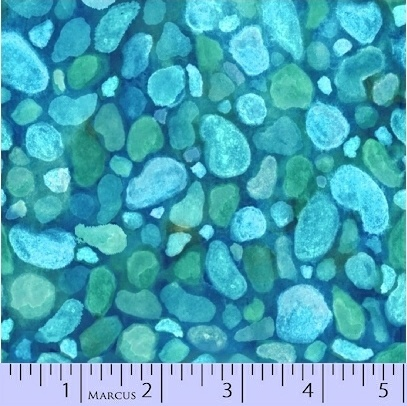Sea Glass fabrics

Beautiful blues and green colors of the sea fabrics, great for a beachy quilt, pillows or bag.

 table div table+table+table+table+table+table div table{width:100%;padding:0}table div table+table+table+table+table+table div table img{width:96.23%;padding:0;float:none}table div table+table+table+table+table+table div table td{width:100%;padding:0 1.88% 18px}/* styles */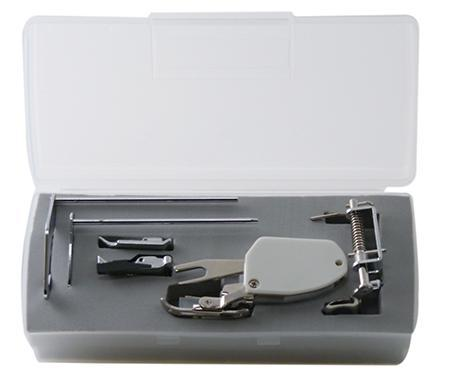New Goodies!

1/4" foot, walking foot and darning foot for a low shank machine in a case.
English Paper Piecing papers and templates for cutting from Sue Daley.
Pigma Pen with nub tip

 table div table+table+table+table+table+table+table+table+table div table{width:100%;padding:0}table div table+table+table+table+table+table+table+table+table div table img{width:96.23%;padding:0;float:none}table div table+table+table+table+table+table+table+table+table div table td{width:100%;padding:0 1.88% 18px}/* styles */Select Accuquilt GO! Dies on Sale!

through 1/31/2019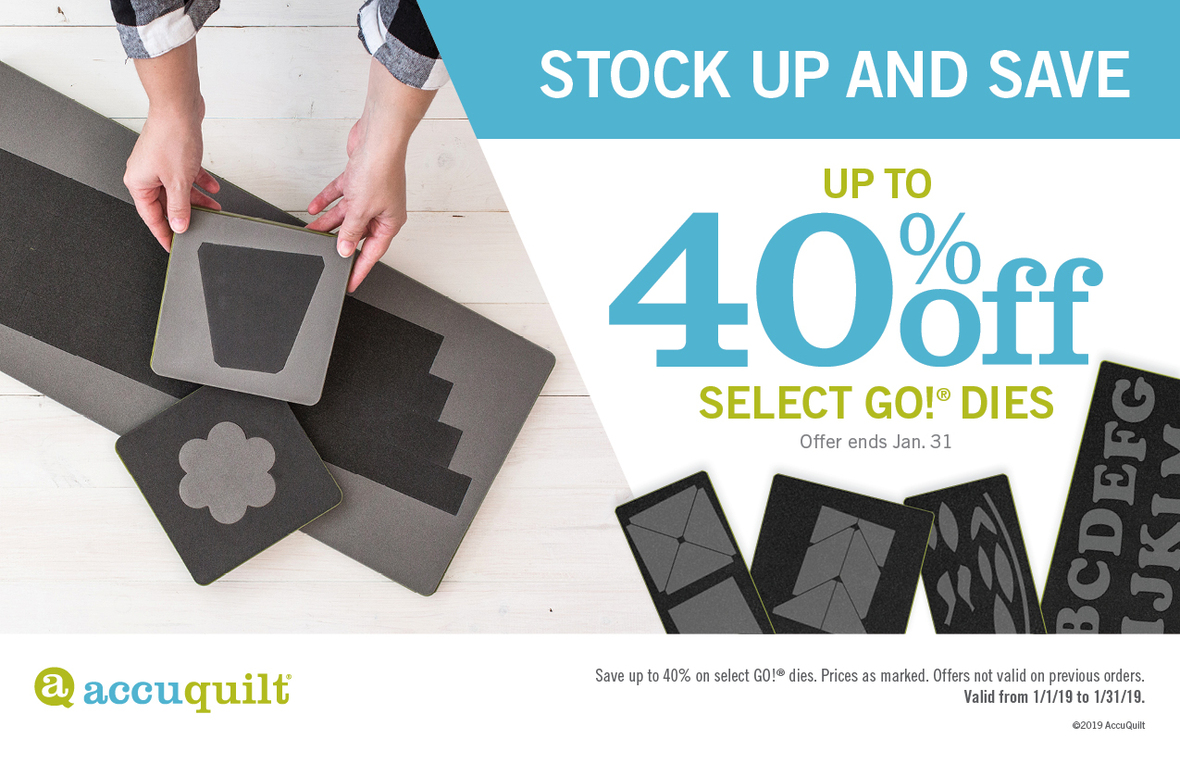table div table+table+table+table+table+table+table+table+table+table+table+table div table{width:100%;padding:0}table div table+table+table+table+table+table+table+table+table+table+table+table div table img{width:96.23%;padding:0;float:none}table div table+table+table+table+table+table+table+table+table+table+table+table div table td{width:100%;padding:0 1.88% 18px}/* styles */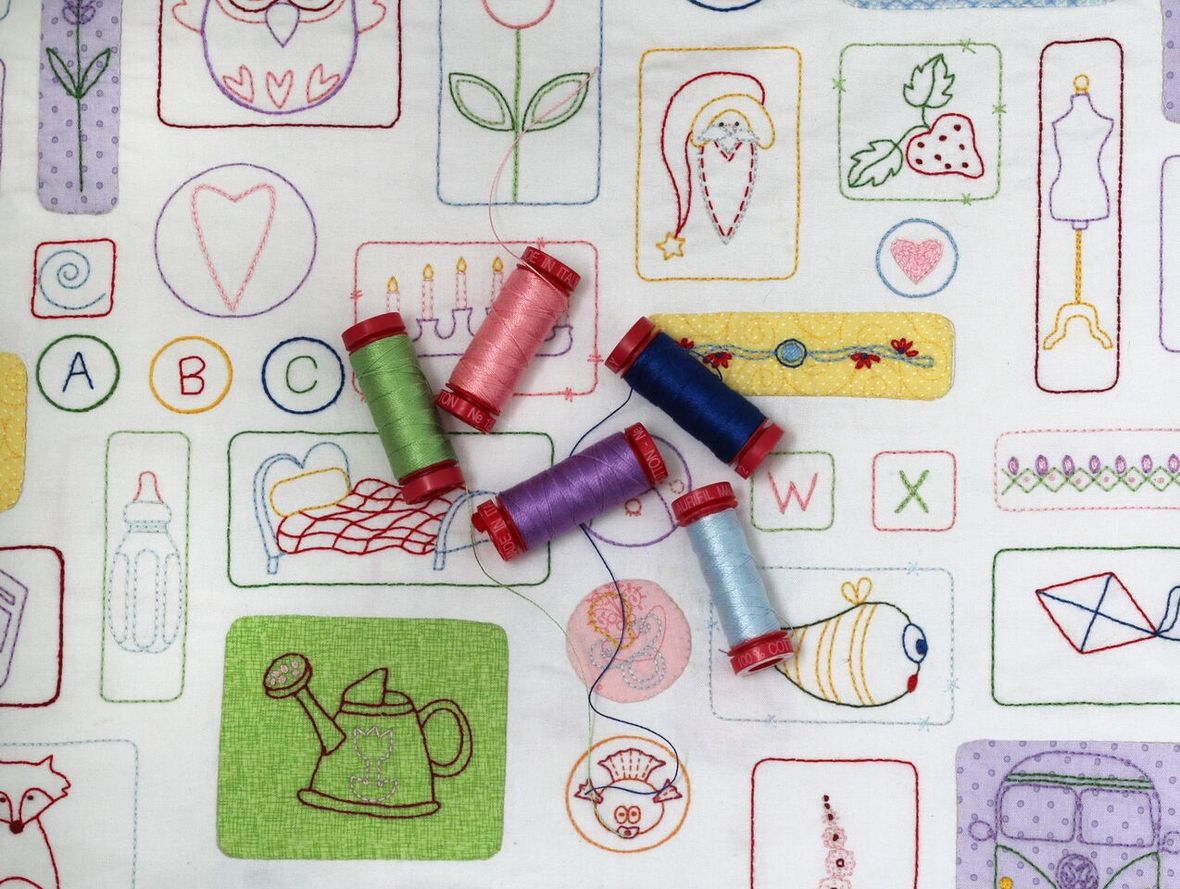Stitch Therapy 365

It's not too late!
We have more panels and thread kits on order AND...
your 365 days start when you get signed up, so no playing catch up, just a little stitching every day.

 table div table+table+table+table+table+table+table+table+table+table+table+table+table+table div table{width:100%;padding:0}table div table+table+table+table+table+table+table+table+table+table+table+table+table+table div table img{width:96.23%;padding:0;float:none}table div table+table+table+table+table+table+table+table+table+table+table+table+table+table div table td{width:100%;padding:0 1.88% 18px}/* styles */Next PMS Night is Friday, January 18

6pm to midnight
Treat yourself to a night of food, fabric and friends!

 table div table+table+table+table+table+table+table+table+table+table+table+table+table+table+table+table+table div table{width:100%;padding:0}table div table+table+table+table+table+table+table+table+table+table+table+table+table+table+table+table+table div table img{width:96.23%;padding:0;float:none}table div table+table+table+table+table+table+table+table+table+table+table+table+table+table+table+table+table div table td{width:100%;padding:0 1.88% 18px}/* styles */Upcoming Classes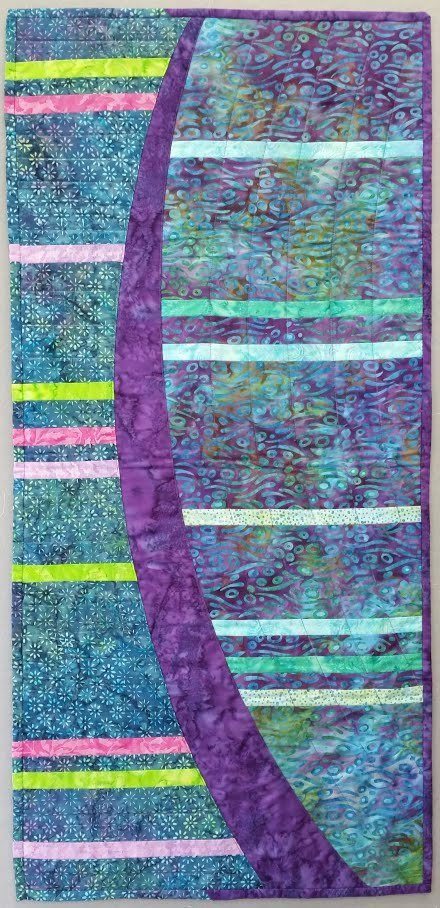New Moon Rising Table Runner

Learn how to use templates, sew curves, practice your cutting 1/4’’ seams, and top stitching.
Class is Saturday, January 12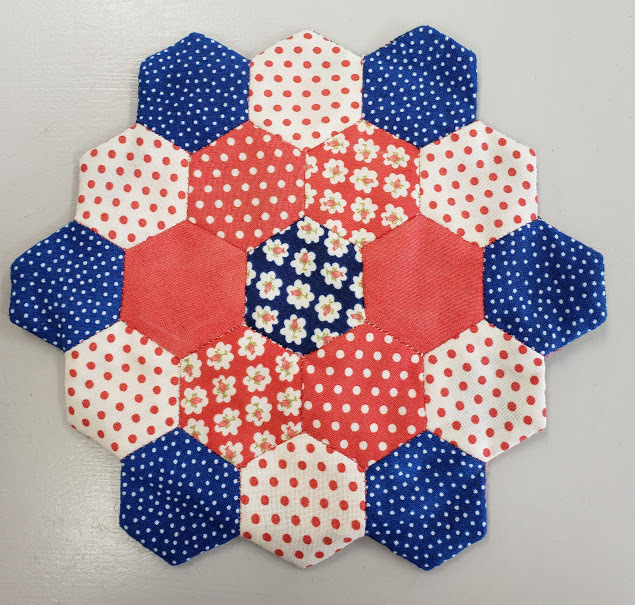English Paper Piecing Gathering

Create projects with the hand sewing technique English Paper Piecing (EPP).
Gathering is Sunday, January 13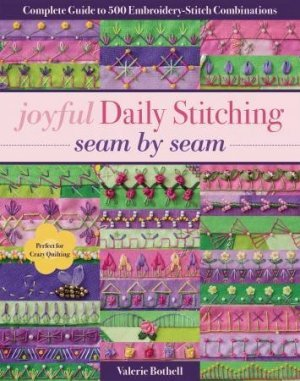Twilight Stitchers

Working from JOYFUL STITCHING book…..we continue on to learn/refresh new stitches.
Anytime is a good time to join in.
Basics will be covered in each class and the skills learned in one fancy stitch or combination will circle back around to previous classes.
Class is Wednesday, January 16, 6pm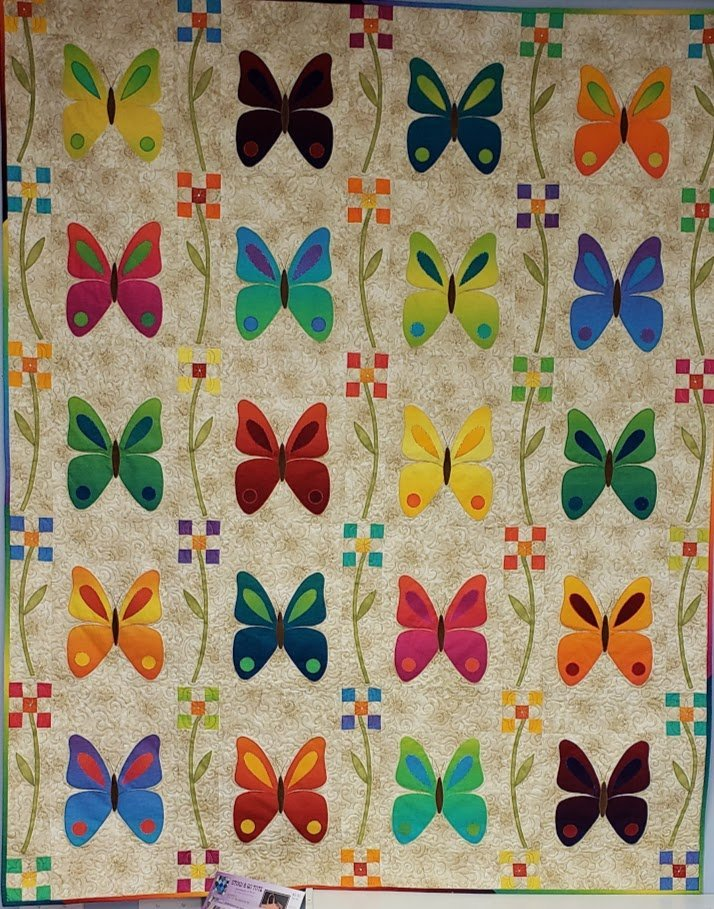GO! Butterfly Patch

You will be amazed at how quickly this quilt goes together when you cut your pieces with the Accuquilt GO! Shop machine and dies available for class use.
Class is Thursday, January 17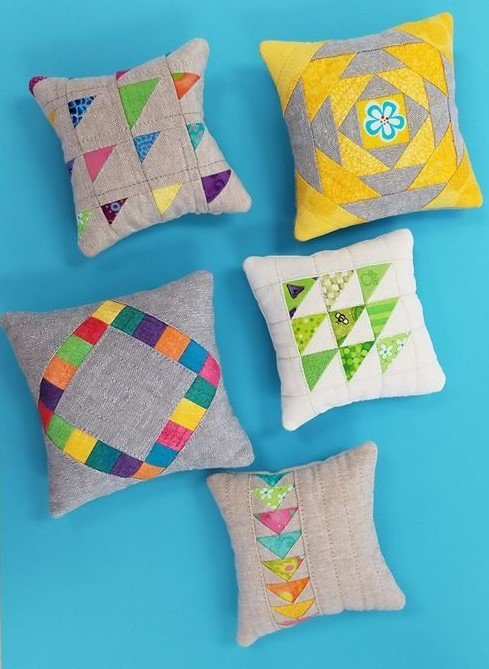Plenty of Pincushions

Such a wonderful accessory, you can never have to many pincushions.
They are useful, decorative and always a welcome gift.
So if you have ever wanted to learn and try some tiny piecing this is your chance
Class is Sunday, January 20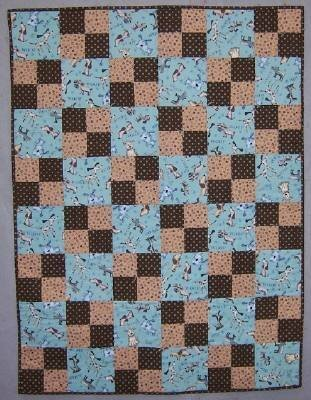Beginning Quilt Making

Small class size insures individual attention.
Daytime or Evening Class available
5 week class, starts Monday, January 21

And there's more!

 table div table+table+table+table+table+table+table+table+table+table+table+table+table+table+table+table+table+table+table+table+table+table+table+table+table+table+table+table+table+table div table{width:100%;padding:0}table div table+table+table+table+table+table+table+table+table+table+table+table+table+table+table+table+table+table+table+table+table+table+table+table+table+table+table+table+table+table div table img{width:96.23%;padding:0;float:none}table div table+table+table+table+table+table+table+table+table+table+table+table+table+table+table+table+table+table+table+table+table+table+table+table+table+table+table+table+table+table div table td{width:100%;padding:0 1.88% 18px}/* styles */Open 7 days a week for your convenience!

Monday through Saturday, 10am to 5pm
Sunday 12pm to 4pm

See you soon!

 table div table+table+table+table+table+table+table+table+table+table+table+table+table+table+table+table+table+table+table+table+table+table+table+table+table+table+table+table+table+table+table+table div table{width:100%;padding:0}table div table+table+table+table+table+table+table+table+table+table+table+table+table+table+table+table+table+table+table+table+table+table+table+table+table+table+table+table+table+table+table+table div table img{width:96.23%;padding:0;float:none}table div table+table+table+table+table+table+table+table+table+table+table+table+table+table+table+table+table+table+table+table+table+table+table+table+table+table+table+table+table+table+table+table div table td{width:100%;padding:0 1.88% 18px}/* styles *//* styles */ Tula is so tall! When I get my photo with her at Market, I am just a shrimp beside her, so this time I was taller than her! (check out her boots!)
 table.module-33{width:97.17%;padding:0}table div table+table+table+table+table+table+table+table+table+table+table+table+table+table+table+table+table+table+table+table+table+table+table+table+table+table+table+table+table+table+table+table+table+table div table{width:97.17%;float:none;margin-left:auto;margin-right:auto;padding:0}table div table+table+table+table+table+table+table+table+table+table+table+table+table+table+table+table+table+table+table+table+table+table+table+table+table+table+table+table+table+table+table+table+table+table div table a{border:0 none;text-decoration:none}table div table+table+table+table+table+table+table+table+table+table+table+table+table+table+table+table+table+table+table+table+table+table+table+table+table+table+table+table+table+table+table+table+table+table div table img{width:100%!important;border:0 none;text-decoration:none}table div table+table+table+table+table+table+table+table+table+table+table+table+table+table+table+table+table+table+table+table+table+table+table+table+table+table+table+table+table+table+table+table+table+table div table td{width:100%;padding:0}/* styles */
 Like   Pin   +1